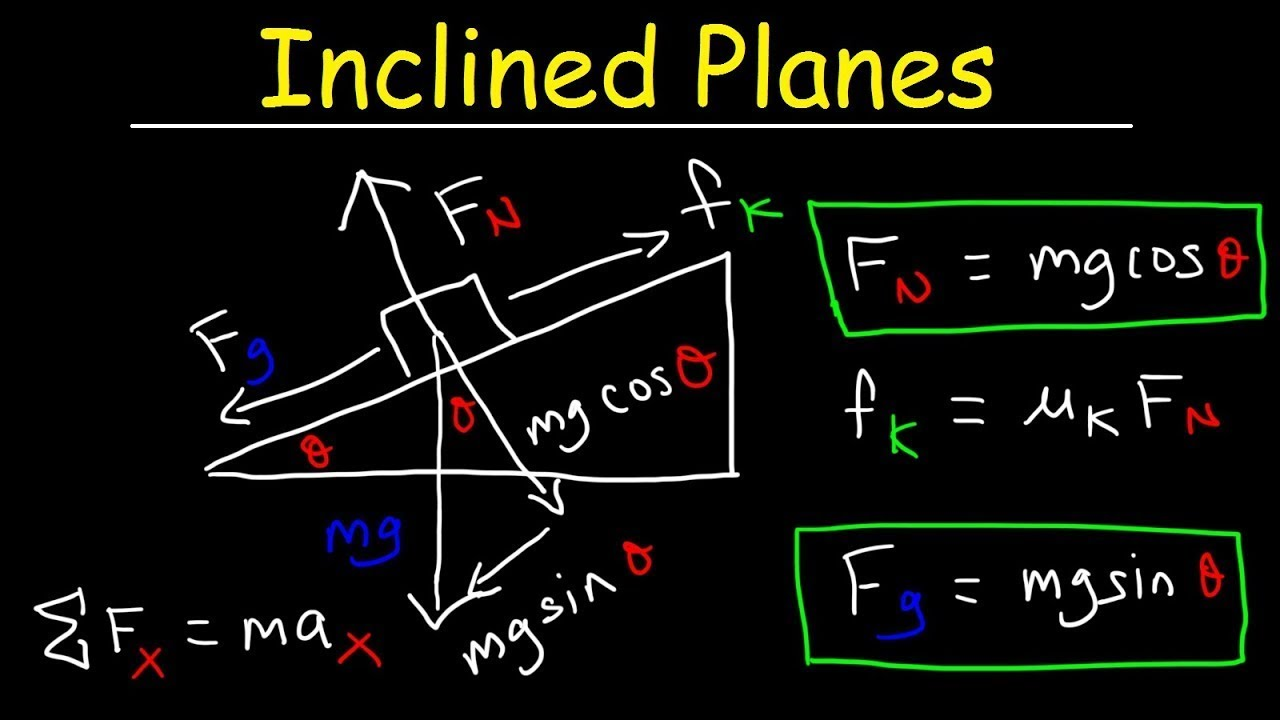1625559420

# Introduction to Inclined Planes

This physics video tutorial provides a basic introduction into inclined planes. It covers the most common equations and formulas that you need to solve inclined plane physics problems. It provides the formulas needed to calculate the normal force, the component force of gravity parallel to the incline, kinetic friction, net force, and the acceleration of the object. It provides the free body diagrams that go with these formulas to solve common problems with newton’s laws of motions.

Full 50 Minute Video: